Question

# 2. Simplify: (n + 2)! (1) n! (2n-1)! (2) (2n + 1)! (2n + 2)! (3)...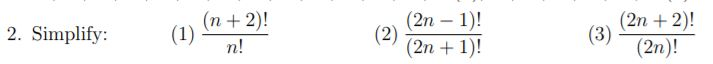2. Simplify: (n + 2)! (1) n! (2n-1)! (2) (2n + 1)! (2n + 2)! (3) (2n)!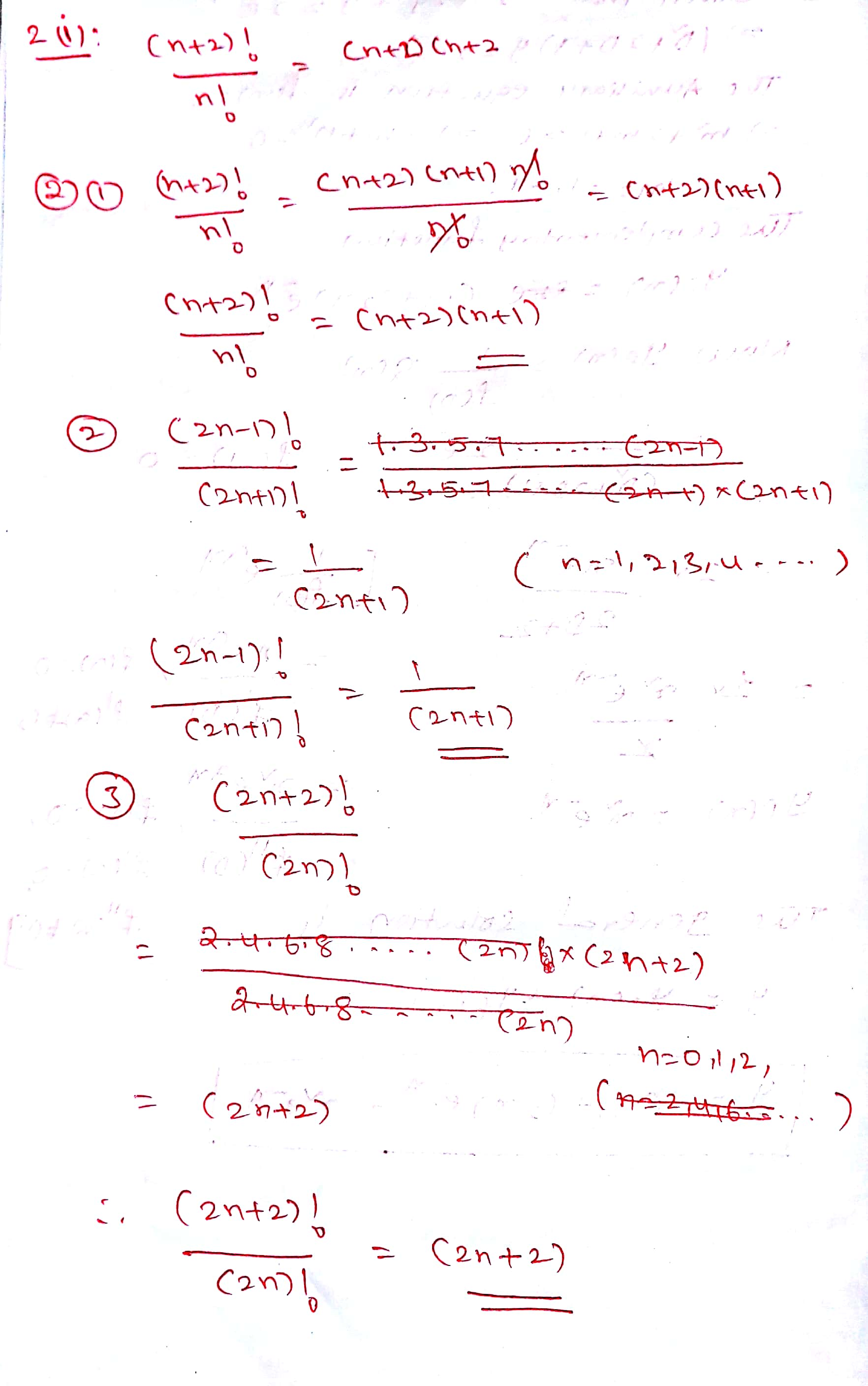#### Earn Coins

Coins can be redeemed for fabulous gifts.

Similar Homework Help Questions
• ### 1. Simplify each expression, for n = 5 a) 8n + 2 b) n2 - 2n+n...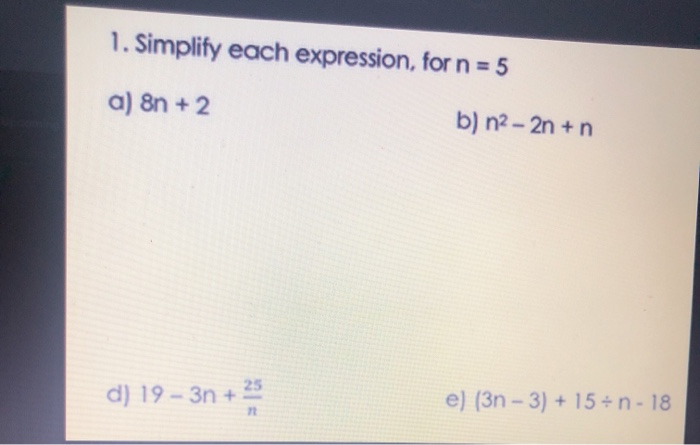1. Simplify each expression, for n = 5 a) 8n + 2 b) n2 - 2n+n d) 19 - 3n + e) (3n - 3) + 15-n-18

• ### Lim n approaches infinity# 6/n((2n)/3 + (5n(n+1))/(2n) - (4n(n+1)(2n+1))/(6n^2))=#?

lim n approaches infinity [6/n(2n/3 + 5n(n+1)/2n - 4n(n+1)(2n+1)/6n^2)]=?

• ### Find a Maclaurin series for f(x). (Use (2n)! —for 1:3:5... (2n – 3).) 2"n!(2n-1) X Rx)...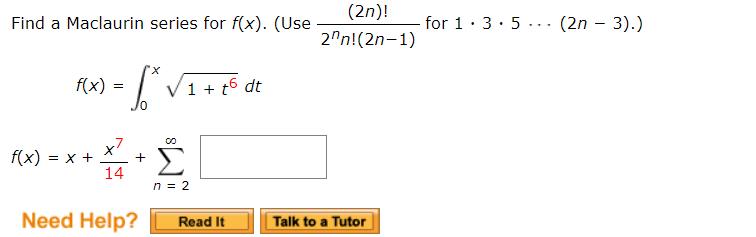Find a Maclaurin series for f(x). (Use (2n)! —for 1:3:5... (2n – 3).) 2"n!(2n-1) X Rx) = (* V1 +48 dt . -*** * 3 n = 2 Need Help? Read It Talk to a Tutor

• ### Find ( ∏3^n−2 )( ∑n(2n−1)) for n = 1 to 3 *the product notation is 3...

Find ( ∏3^n−2 )( ∑n(2n−1)) for n = 1 to 3 *the product notation is 3 to 'n' only, -2 is not raised power* Group of answer choices 1650 36 3850 3675

• ### Simplify each expression. Thanks 4n 6 n-3 2n 7) a+4_6 2a +6 2 8) 9) x+5...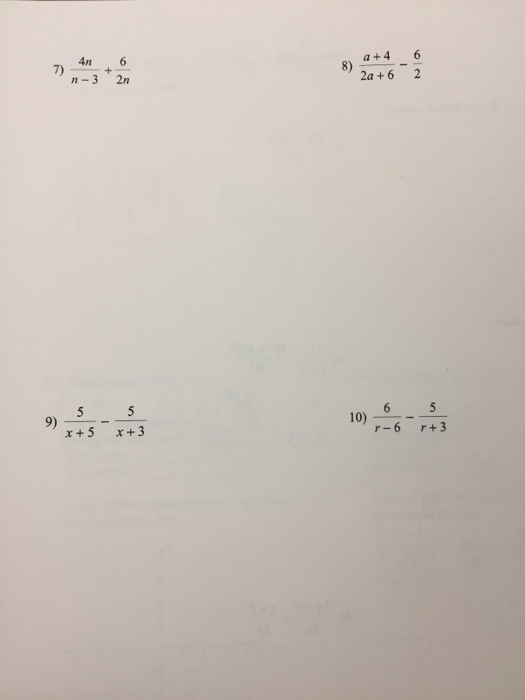Simplify each expression. Thanks 4n 6 n-3 2n 7) a+4_6 2a +6 2 8) 9) x+5 x +3 10) 6 r-6 r+ 3

• ### Prove: without using l'hopital's rule. infinity 2n-1 ln(2) (2n-1) n infinity 2n-1 ln(2) (2n-1) n

Prove: without using l'hopital's rule. infinity 2n-1 ln(2) (2n-1) n infinity 2n-1 ln(2) (2n-1) n

• ### 2n 3. Prove that lim n+on+ 1 2.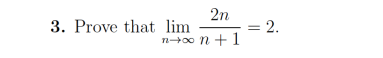2n 3. Prove that lim n+on+ 1 2.

• ### Among the following series, which one does converge conditionally? n=1 Σ(-1)"re-n 1-3-5 3 3-5 3-5.7 1-3-5(2n-1 -(2n) 1.3.5 (2n-1) (-1)" n=1 Σ(-1)"re-n 1-3-5 3 3-5 3-5.7 1-3-5(2n-1 -(...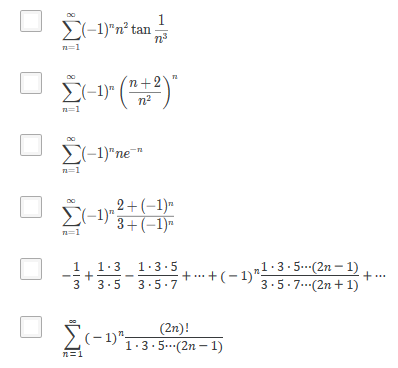Among the following series, which one does converge conditionally? n=1 Σ(-1)"re-n 1-3-5 3 3-5 3-5.7 1-3-5(2n-1 -(2n) 1.3.5 (2n-1) (-1)" n=1 Σ(-1)"re-n 1-3-5 3 3-5 3-5.7 1-3-5(2n-1 -(2n) 1.3.5 (2n-1) (-1)"

• ### Some useful identities Using (2.3), we have n2n-1 n2n-1 + n(n 1)2"-2 non-1 + n(n-1)(n-2)2n-3 +...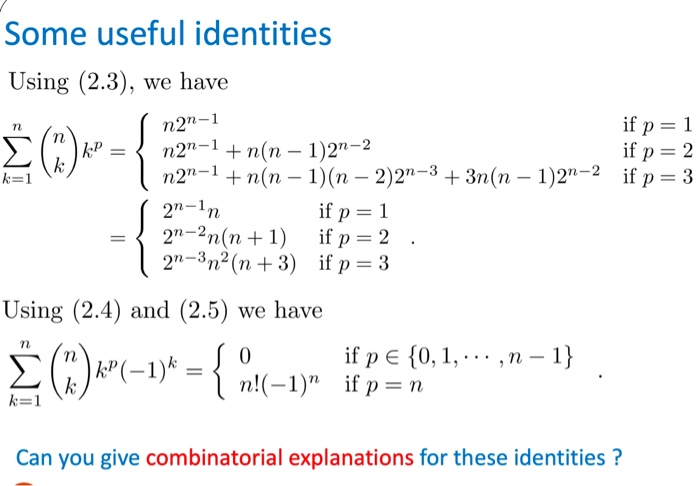Some useful identities Using (2.3), we have n2n-1 n2n-1 + n(n 1)2"-2 non-1 + n(n-1)(n-2)2n-3 + 3n(n-1)2n-2 7n İfp-3 n- 22n(n1) if p 2 2"-3n2(n +3) if p3 Using (2.4) and (2.5) we have 0 ifpe(0, 1, ,n-1} Can you give combinatorial explanations for these identities?

• ### Prove that P2n(0)= (-1)n ((2n-1)!!/(2n)!!) using the generation function and a binomial expansion...

Prove that P2n(0)= (-1)n ((2n-1)!!/(2n)!!) using the generation function and a binomial expansion. Show that (sqrt(pi)(4n-1)/(2gamma(n+1)gamma(3/2-n))=(-1)n-1((2n-3)!!/(2n-2)!!)(4n-1)/2n